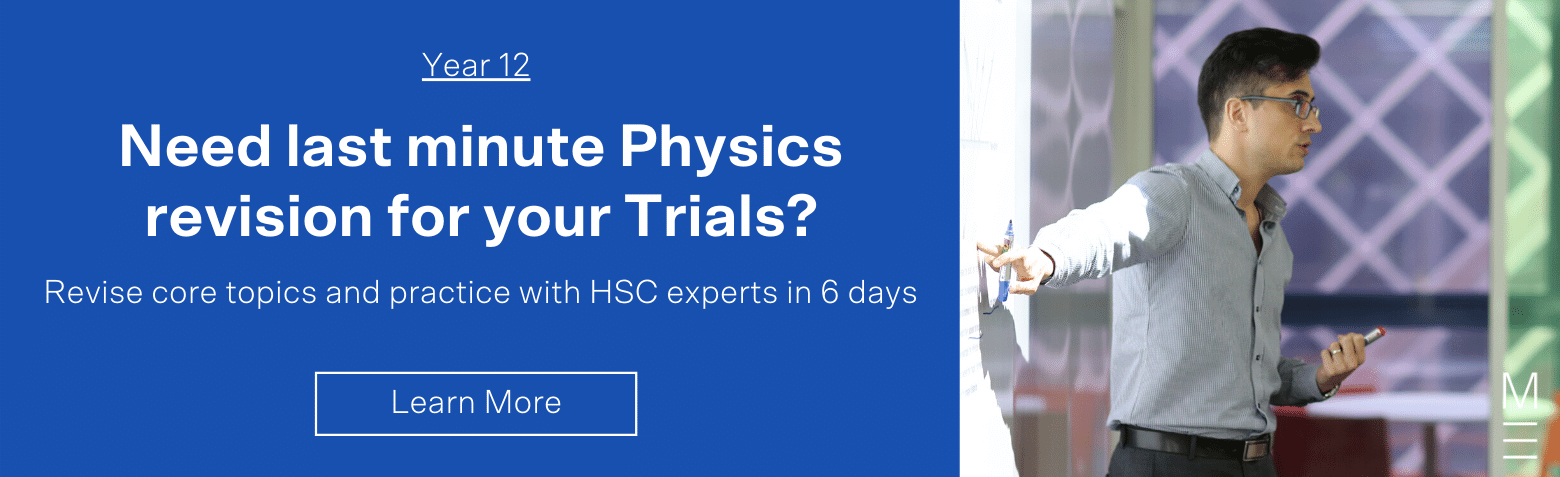# How to answer ‘Explain’ HSC Physics Questions

In this post, the Matrix Science Team show you how to answer "Explain" Physics questions.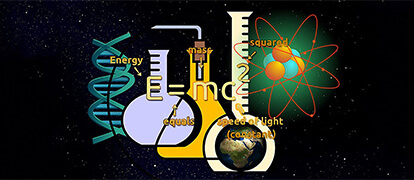In the 2014 HSC Physics Exam, there were 9 questions that involved the following key verbs:

• Identify (Q21, Q25a)
• Outline (Q25b)
• Explain (Q23, Q27a, Q30a, Q31)
• How does |one thing| affect |another thing| (Q22, Q29)

See the complete Glossary of Key Words.

Did you know that most students lose a mark or two when answering these questions? If you were to lose one mark for each ‘Explain’ question in 2014, it would equate to at least 4 marks! The seriousness of this problem is highlighted by the HSC Marking Centre every year in ‘HSC Notes from the Marking Centre

Students can generally benefit by correct interpretation of questions

Let’s read the ‘Common Mistakes’ that students make in Part (B):

Examiners provide reports after each HSC exam that indicates areas of strengths and weaknesses in student responses. From these common mistakes, it is clear that students generally can benefit by:

1. More precise and scientific use of terminology, especially that specified in the syllabus;
2. More precision with graphs and labelling of diagrams;
3. Avoiding answers that are too long or detailed or that use ambiguous terms;
4. Correct interpretation of questions, e.g. Listing instead of describing, or giving a description not an explanation;
5. Answering in specific terms, not generalities;
6. Ensuring all parts of multi-part questions are attempted;
7. Being able to clearly describe procedures, apparatus, etc. for mandatory practical activities as a first-hand experience.

The 6 most common mistakes HSC Physics students make in exams article lists several additional common mistakes that are frequently made in exams, such as insufficient workings for calculations and mistakes made when drawing a line of best fit.

## How to Answer ‘Explain’ Questions

‘Explain’ is an extremely common key verb used for 2-3 mark exam questions. The Board of Studies Glossary of Key Words defines ‘Explain’ as “relate cause and effect; make the relationships between things evident; provide why and/or how.”

Many of the questions in Physics are about explaining the physical link between cause and effect. Let’s look at ‘explain’ questions from 2014 HSC Physics Exam Paper:

1. 2014 HSC Q23 (3 marks): Explain how the current in the wire loop affects the straight conductor (8 lines)
2. 2014 HSC Q25b (3 marks): Explain how the motor effect is used in an AC motor (6 lines)

‘Explain’ questions tend to have the following structure:

1. Explain how/why |finish point| when/ using |start point|
2. Explain using/how |start point| affects/causes |finish point|

When answering questions involving ‘Explain’, students should consider the following structure: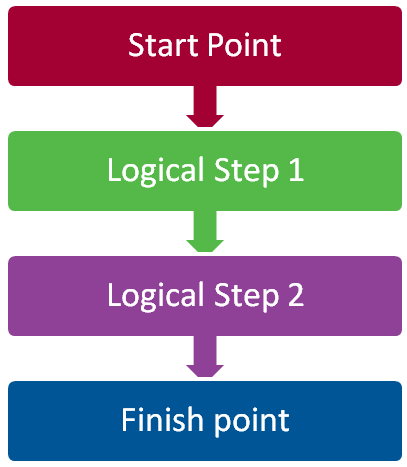Explain links a start point to a conclusion using scientific arguments and needs a sequential flow of logic and from start point to finish point. Let’s answer an explain question using the structure outlined above.

QUESTION (2014 HSC Q25b – 3 marks):
Explain how the motor effect is used in an AC motor.

Step 1: Identify the start and finish point
Explain how |the motor effect |is used in an AC motor|

Start to Finish Point: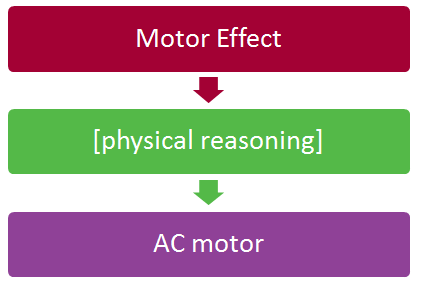OR

Finish to Start Point: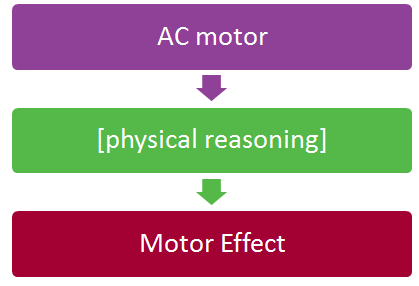Step 2: Use a relevant law of Physics to provide physical reasoning
It usually doesn’t matter which end point  you choose to be the |start point| and |finish point|, as long as there’s a logical argument linking one to the other.1. The motor effect is when a current-carrying wire is placed in a magnetic field experiences a force in a certain direction due to the interacting magnetic field.
2. If this wire loop rotates around an axis, the motor effect can rotate the loop by a maximum of 180 degrees. If the direction of current is changed at this point, the direction of force is reversed.
3. A rotating wire loop carrying alternating current in a magnetic field can thus undergo continuous rotations due to the motor effect.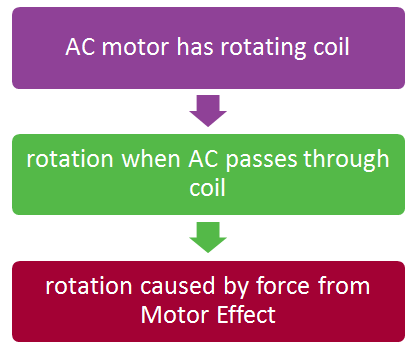1. An AC motor uses a wire loop carrying AC in a magnetic field which undergoes continuous rotations due to magnetic force.
2. This is because the wire loop rotates around an axis, and the motor effect rotates the loop by a maximum of 180 degrees. If the direction of current is changed at this point, the direction of force is reversed.
3. The motor effect causes this motion because it is the effect that when a current-carrying wire is placed in a magnetic field, it experiences a force in a certain direction due to the interacting magnetic field.

This is not as natural in this question, but it is still a valid explanation response. In other questions there is no difference.

Notice the logical links between the points in Answer 1 are which results in or therefore (⇒, ∴), and the links between the points in Answer 2 are caused by or because or since (⇐,∴). Both answers thus feature a logical argument from start to finish.

## Here are 3 Student Sample ‘Explain’ Responses Ranging from Band 1 to Band 6.

Band 1/2: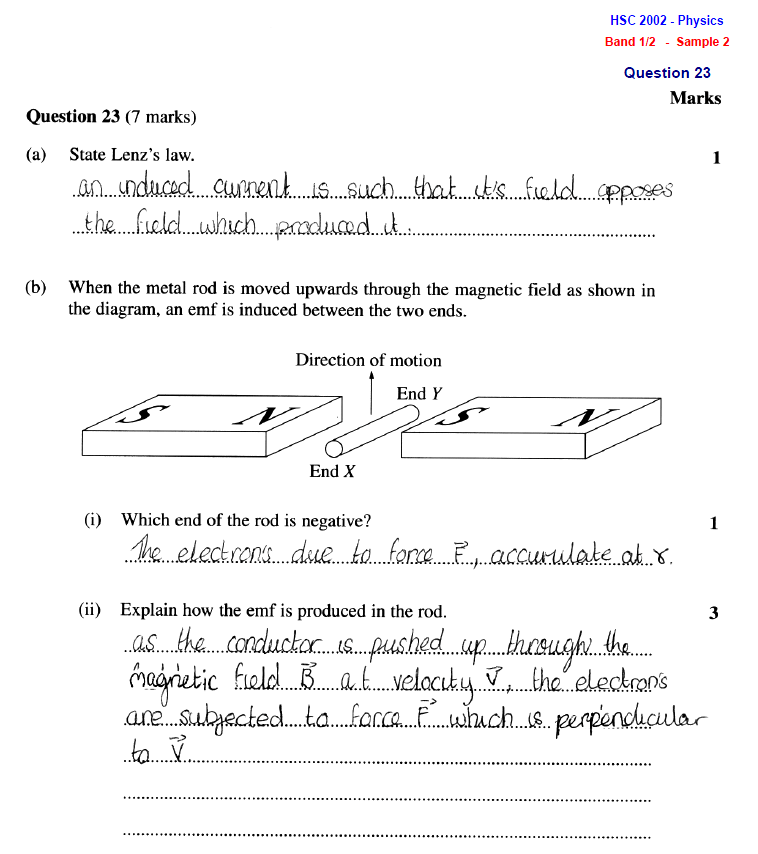Band 3/4: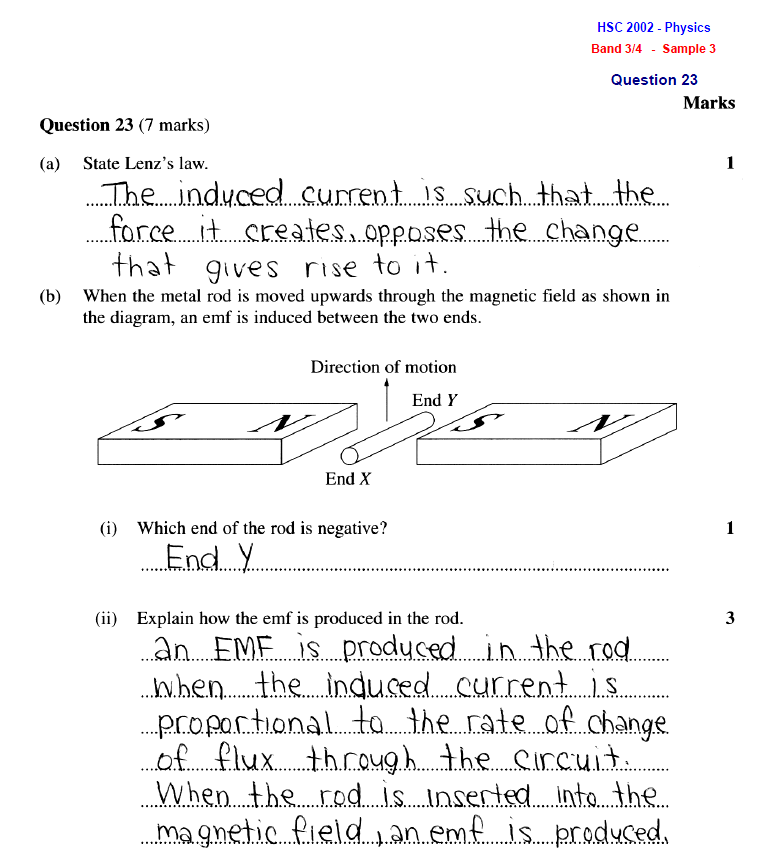Band 5/6: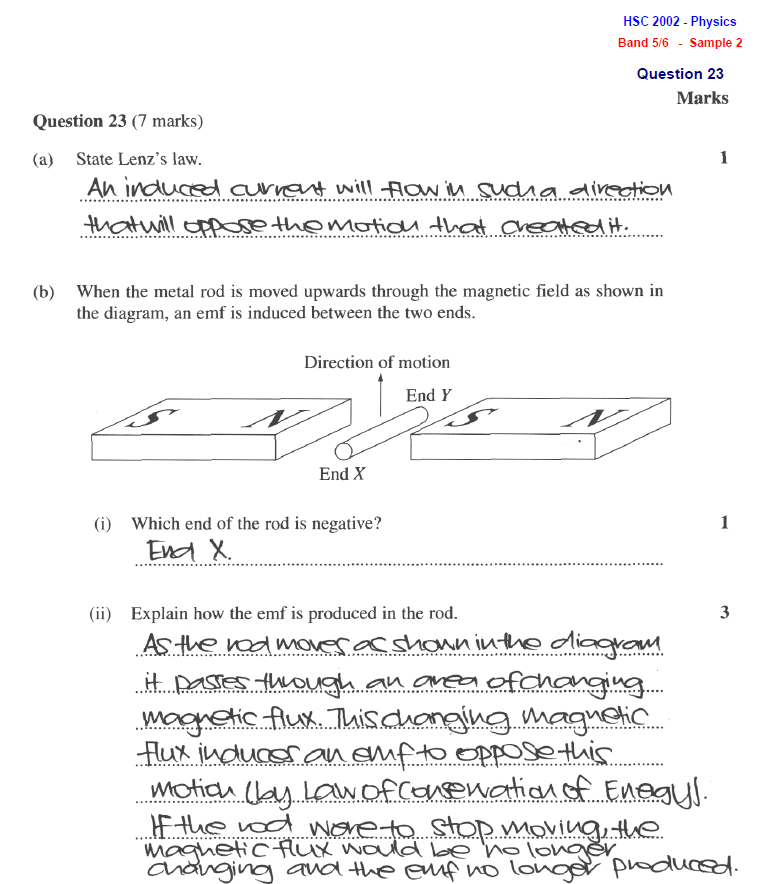## Need a hand improving your Physics rank?

Our HSC Physics Trial Exam Prep Course will get you on top of your marks in just 9 days. Learn more.